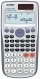# Equation 11

Solve equation:
$0=y-1,2.\left(y-1,5\right)$

y =  9

### Step-by-step explanation:

0=y-1,2•(y-1,5)

0=y-1.2•(y-1.5)

0.2y = 1.8

y = 9

Our simple equation calculator calculates it.Did you find an error or inaccuracy? Feel free to write us. Thank you!Tips to related online calculators
Do you have a linear equation or system of equations and looking for its solution? Or do you have a quadratic equation?

## Related math problems and questions:

• EquationSolve equation and check the result: 1.4x - 3/2 + x - 9,8 = x + 0,4/3 - 7 + 1,6/6
• Equation 29Solve next equation: 2 ( 2x + 3 ) = 8 ( 1 - x) -5 ( x -2 )
• Simple equationSolve the following simple equation: 2. (4x + 3) = 2-5. (1-x)
• Find xSolve: if 2(x-1)=14, then x= (solve an equation with one unknown)
• Sorting ASCWhich group of decimals is in order from least to greatest? A) 1.04, 0.39, 0.8, 2.1, 0.09 B) 2.1, 1.04, 0.39, 0.8, 0.09 C) 0.09, 0.39, 0.8, 1.04, 2.1 D) 0.09, 0.39, 0.8, 2.1, 1.04
• Simple equation 9Solve the following equation: -8y+5=-9y+9
• Fraction of a NumberIf 1/2 of 1/3 of 1/4 of 1/5 of a number is 2.5. what is the number?
• Without 2Without multipying, tell whether the product 0.644 x 0.25 will be greater than 1 or less than 1? Explane how you know. Then find the product.
• Ratio6 numbers are in the ratio 1:5:1:5:5:5. Their sum is 242. What are the numbers?1st blade 2,5 m, 2nd blade. .1.75 m. How many same long pieces of this two blades can be do the biggest? How long is one piece?
• Unknown number 6Determine the unknown number, which is by 1.5 greater than its fourth.
• Linear systemSolve a set of two equations of two unknowns: 1.5x+1.2y=0.6 0.8x-0.2y=2
• Unknown number 6Determine x if 1/6 of x is equal to 2/5 of the number 24.
• Reciprocal equation 3Solve reciprocal equation: 1/2 + 2/3=1/x
• The length 6The length of 12 pipes is 10 1/2 meters. (1) find the length of one pipe (2) also find the length of 7 pipes
• EquationFind x: x + 1/2 = 1/3
• Alice 2Alice is swimming 2.5 hours every Saturday and 3.5 hours every Sunday. How many hours does she swim in 4 weeks?